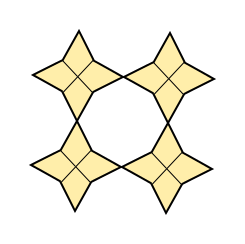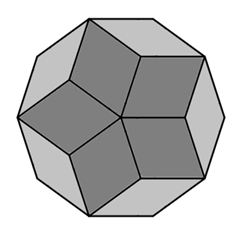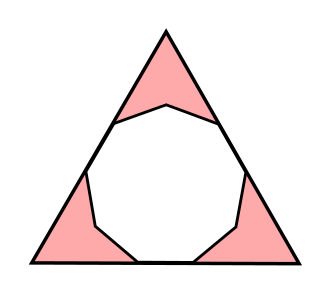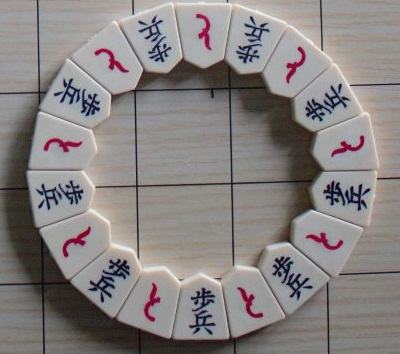#### You may also like### On Time

On a clock the three hands - the second, minute and hour hands - are on the same axis. How often in a 24 hour day will the second hand be parallel to either of the two other hands?### Estimating Angles

How good are you at estimating angles?### LOGO Challenge 1 - Star Square

Can you use LOGO to create this star pattern made from squares. Only basic LOGO knowledge needed.

# Shogi Shapes

##### Age 11 to 14Challenge Level

Can you work out the angles in the pictures below?

On the left, a regular octagon is surrounded by congruent kites. On the right, a regular decagon is divided into two different types of rhombuses.Here are two more polygon puzzles to try.

The first one is a regular nonagon drawn inside an equilateral triangle. The second is also a regular nonagon, with lines joining vertices to each other and to the centre.
Can you work out the angles?The game of Shogi, also known as Japanese Chess, is played with tiles on a 9 by 9 board.
Here is a picture of some Shogi pieces that have been arranged in a pattern:What questions might a mathematician ask?
Here are some that we thought of:
• Is there a quick way to count the number of tiles in the picture?
• What shape are the tiles?
• Which angles can be calculated?
• If we assume that the tiles are symmetrical, there are two pairs of matching angles and one other angle. What is the range of possible sizes of that "lonely" angle?
• Could we make other sets where we need more tiles to go all the way round with no gaps?